# ICSE Class 10 Sample Question Paper Maths 2020 With Answers | Specimen Papers Maths 2020 - Free PDF Download

ICSE Class 10 Maths 2020 Sample Question Paper with Answers can be accessed online and also in a downloadable pdf format at BYJU’S. After completing the ICSE class 10 Maths syllabus students are advised to practise ICSE Maths Specimen Papers 2020 Solved for Class 10 provided at BYJU’S. Students can also revise their solutions of the 2020 ICSE class 10 maths sample question paper which are prepared by our expert faculty.

Answers of these ICSE sample paper maths for class 10 2020 will help the students to resolve their doubts in the identification of the right approach for different types of questions. Practising more sample papers for class 10 maths will improve the confidence level of students as well as their problem-solving skills.

#### ICSE Class 10 Maths 2020 Sample Paper with Solutions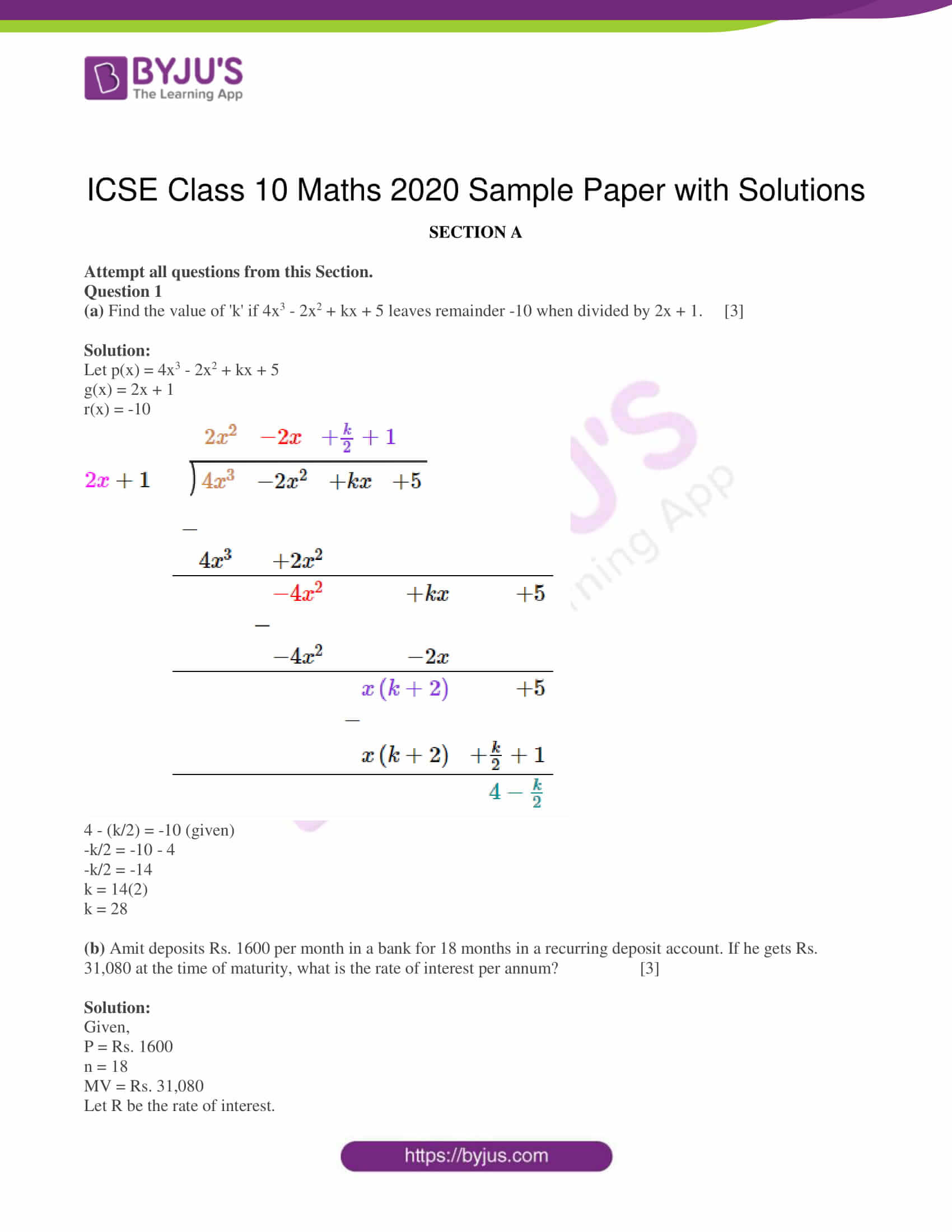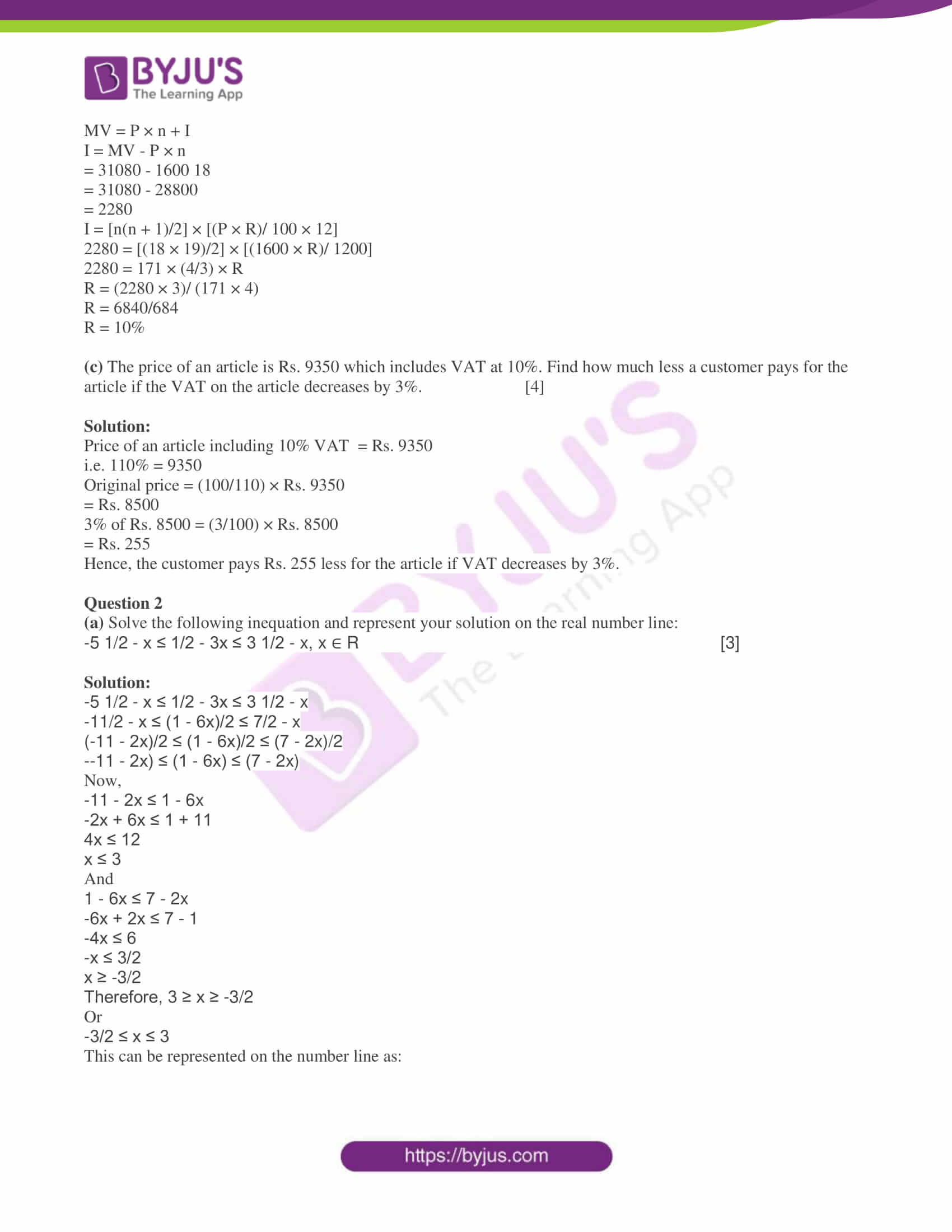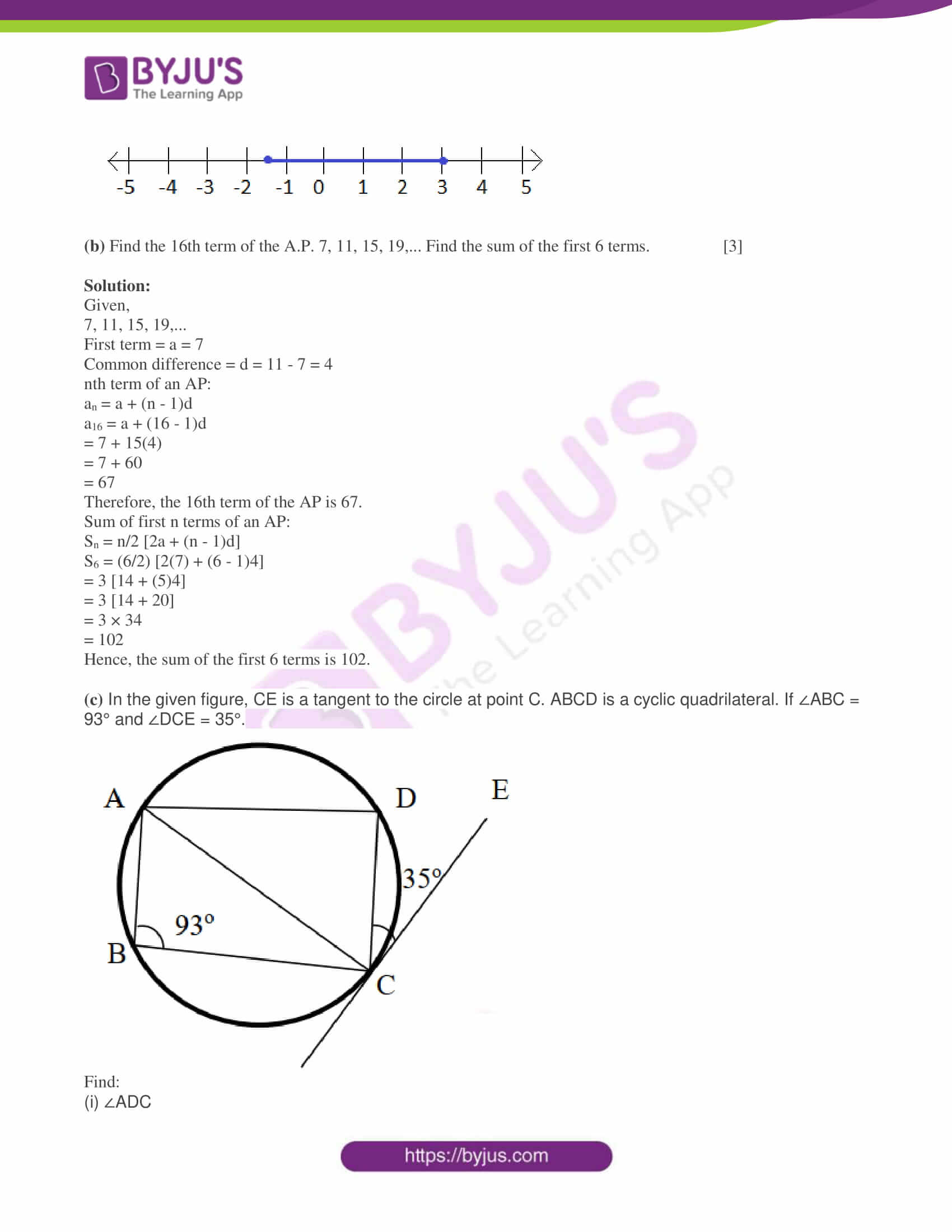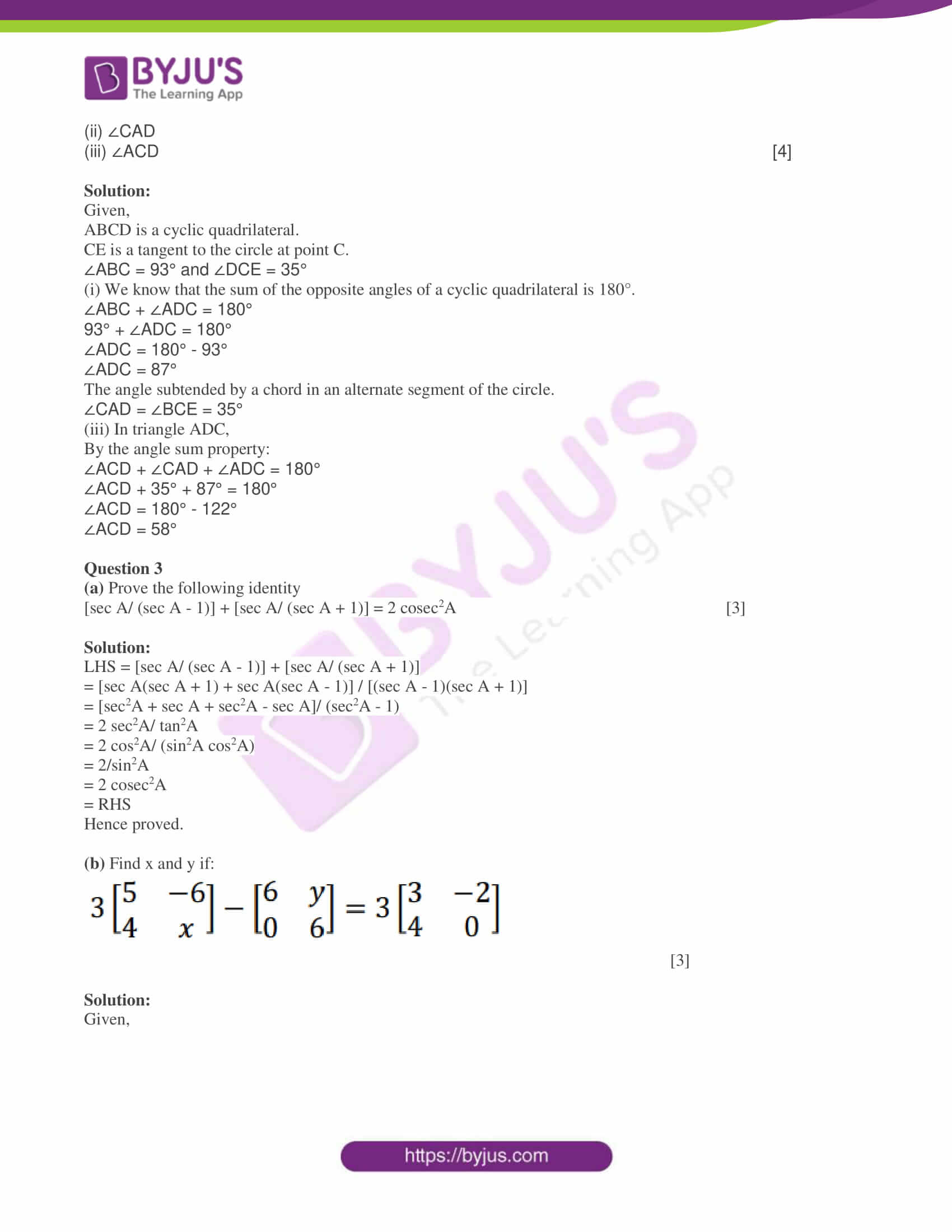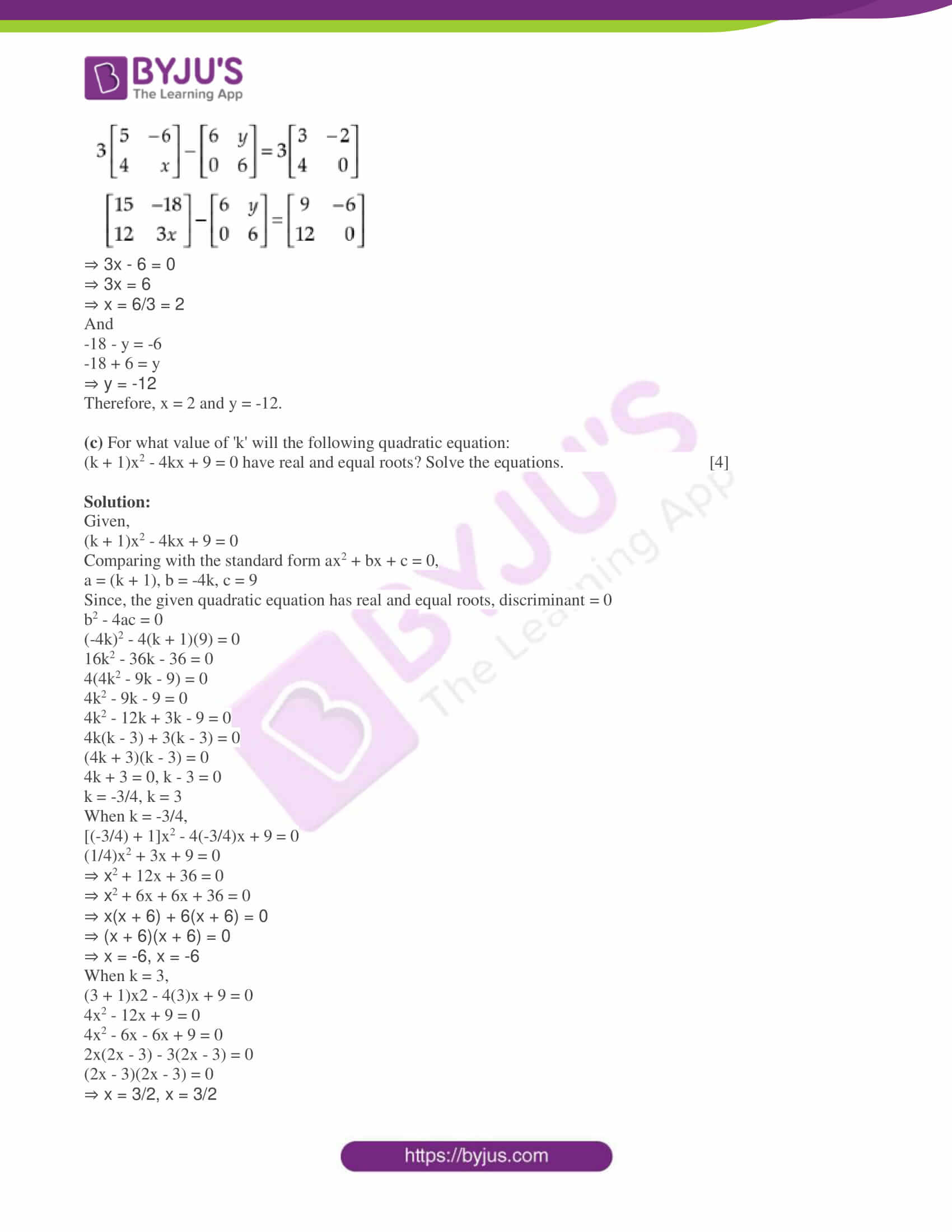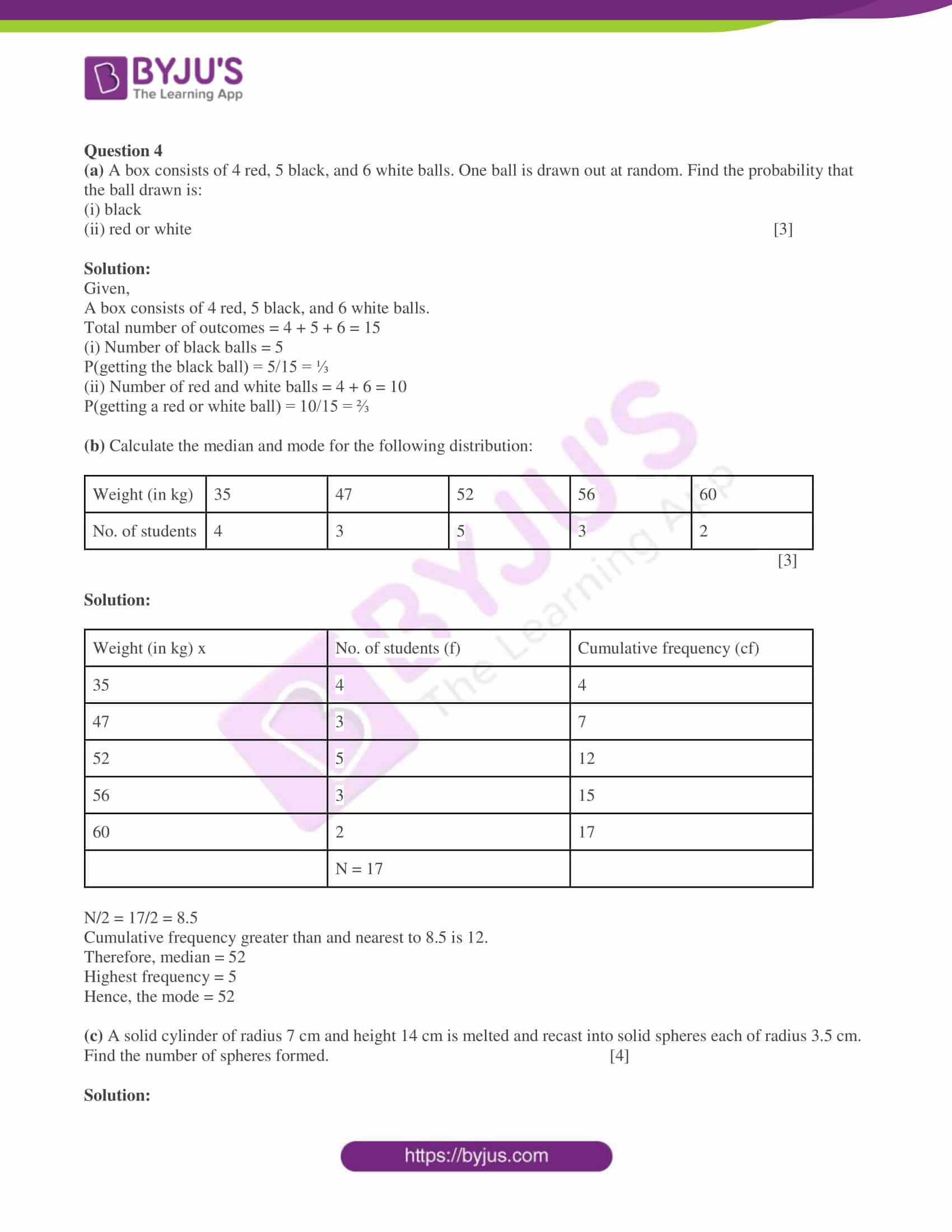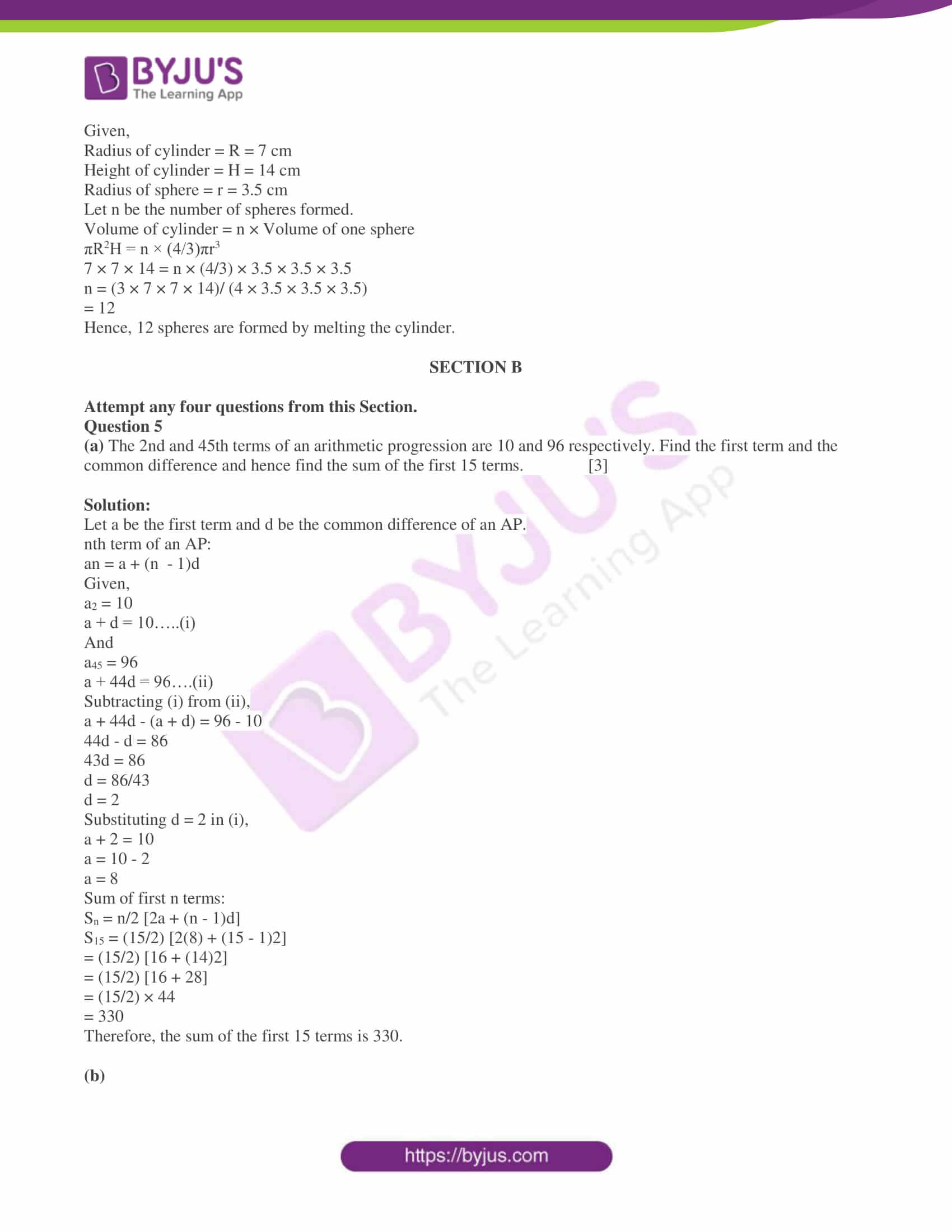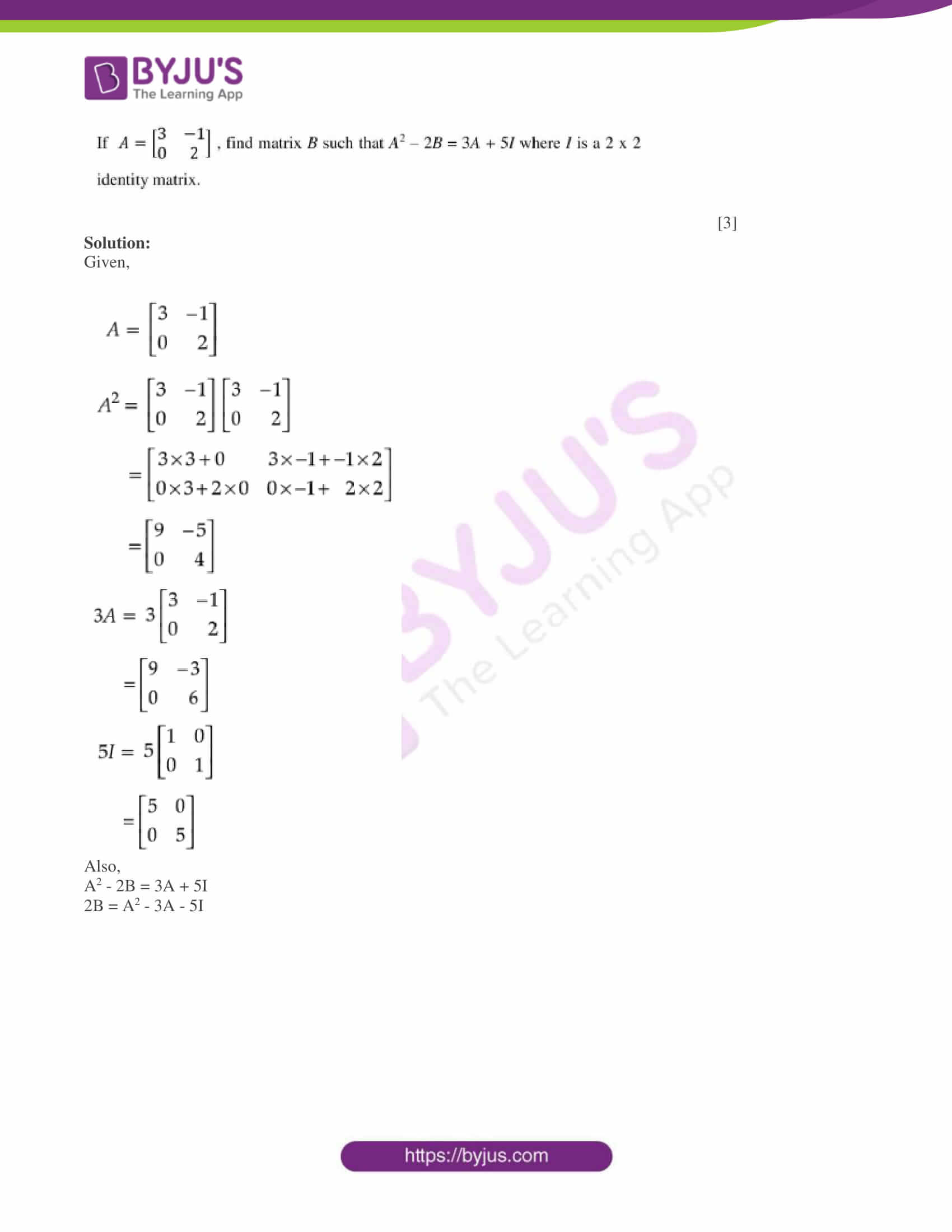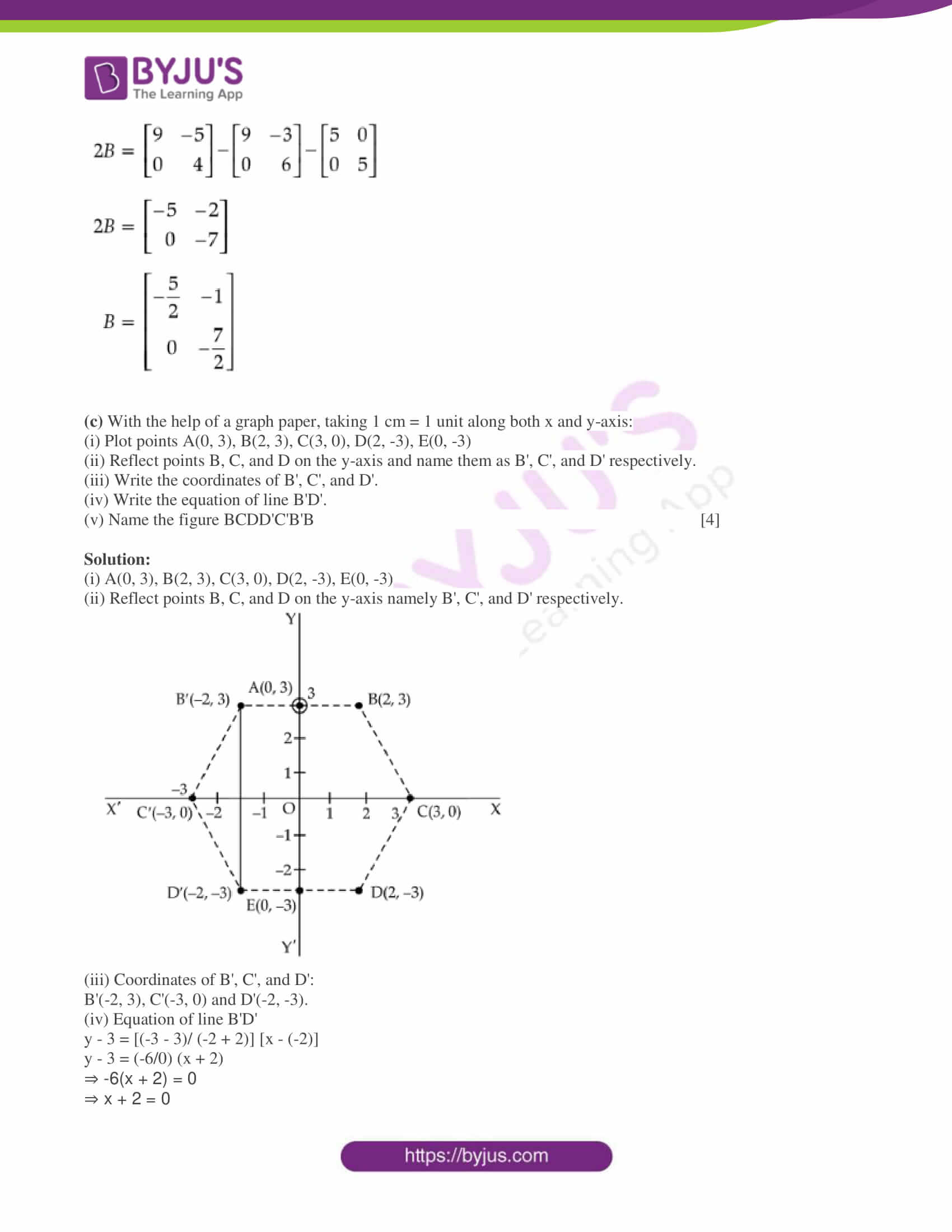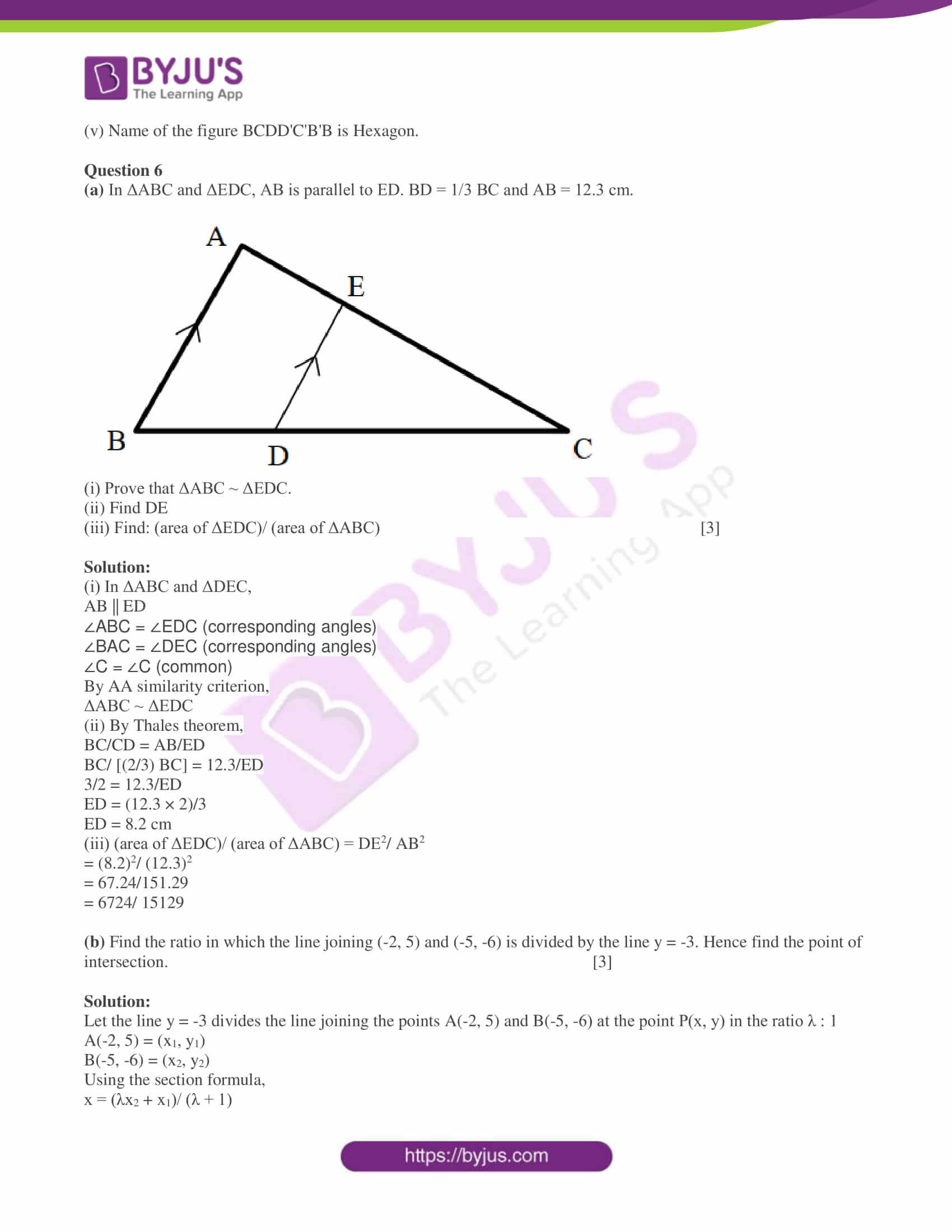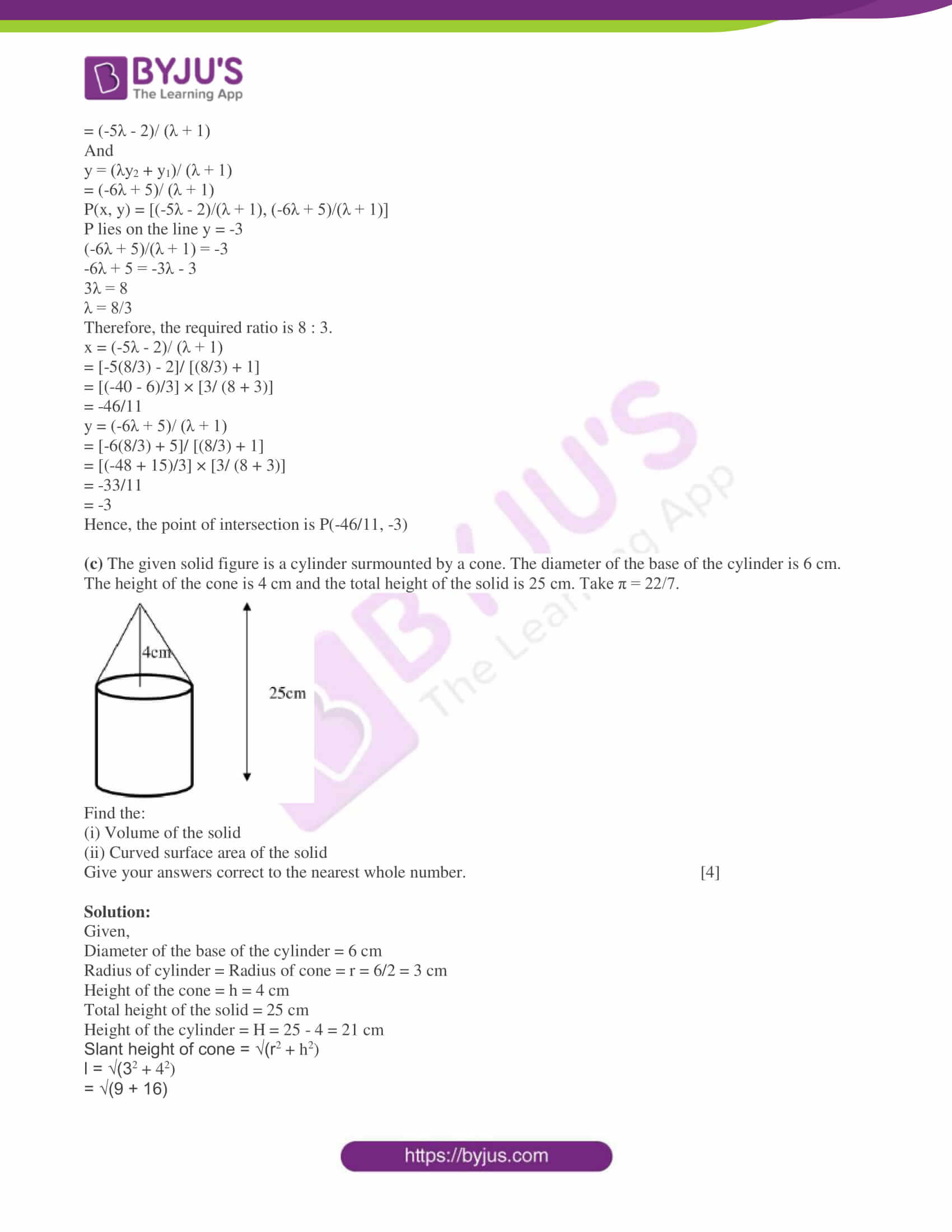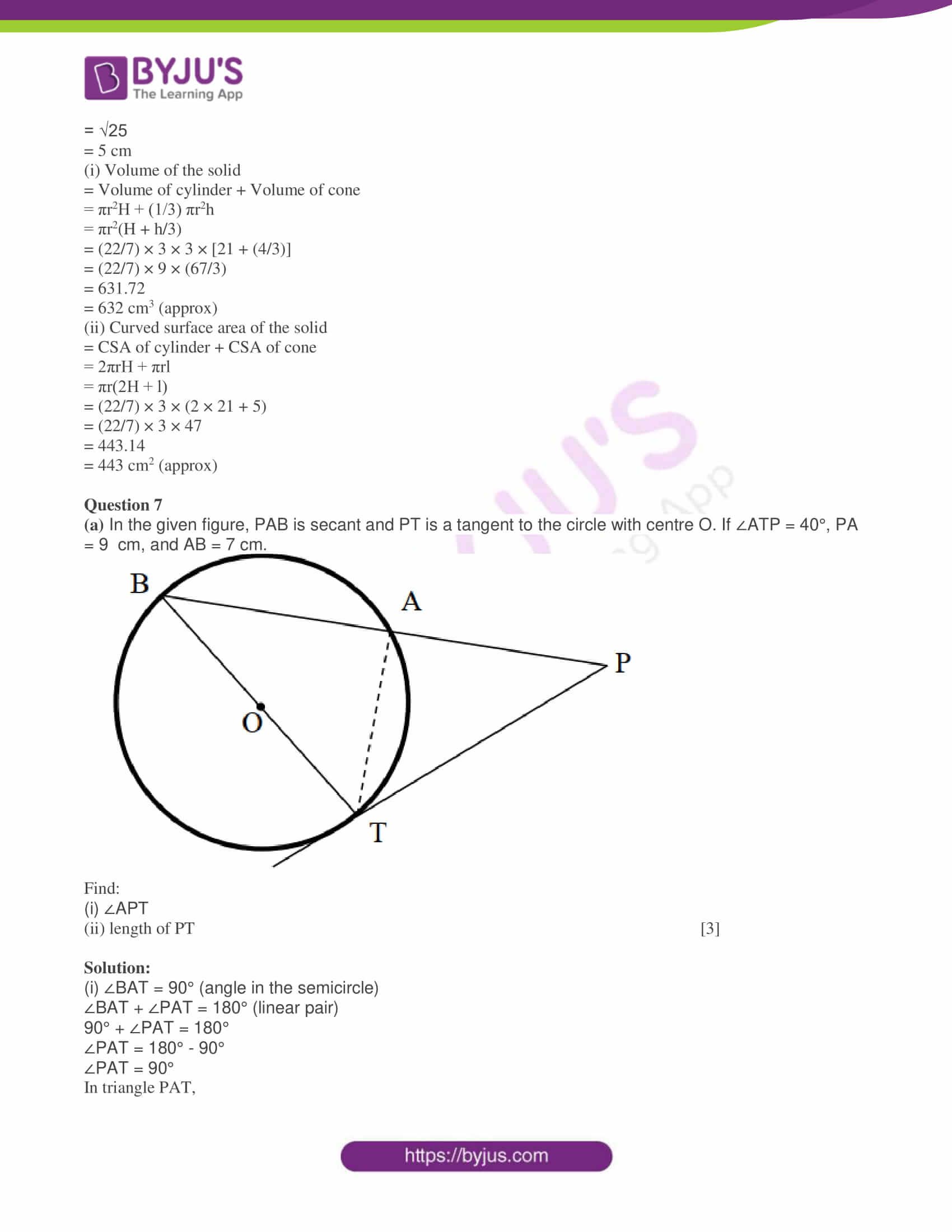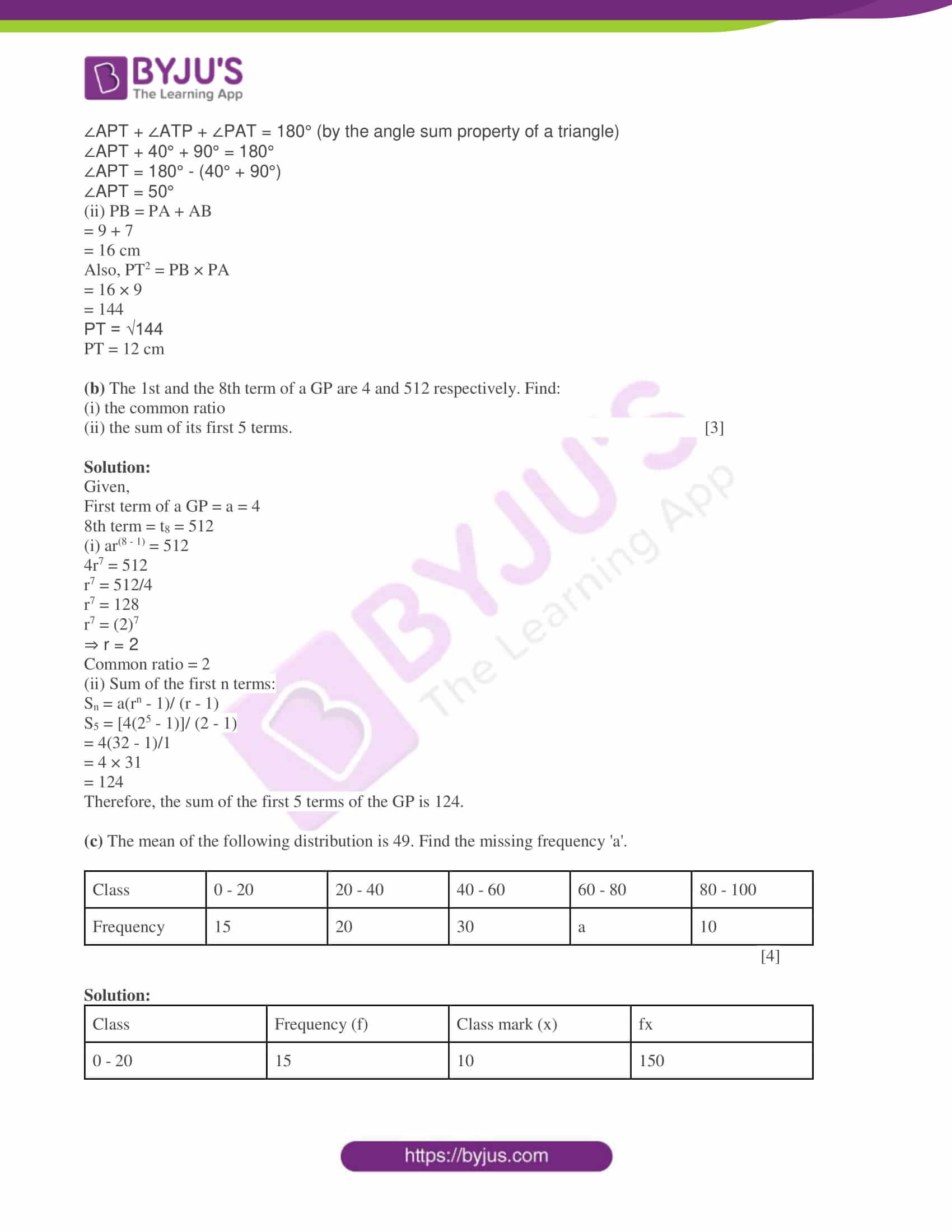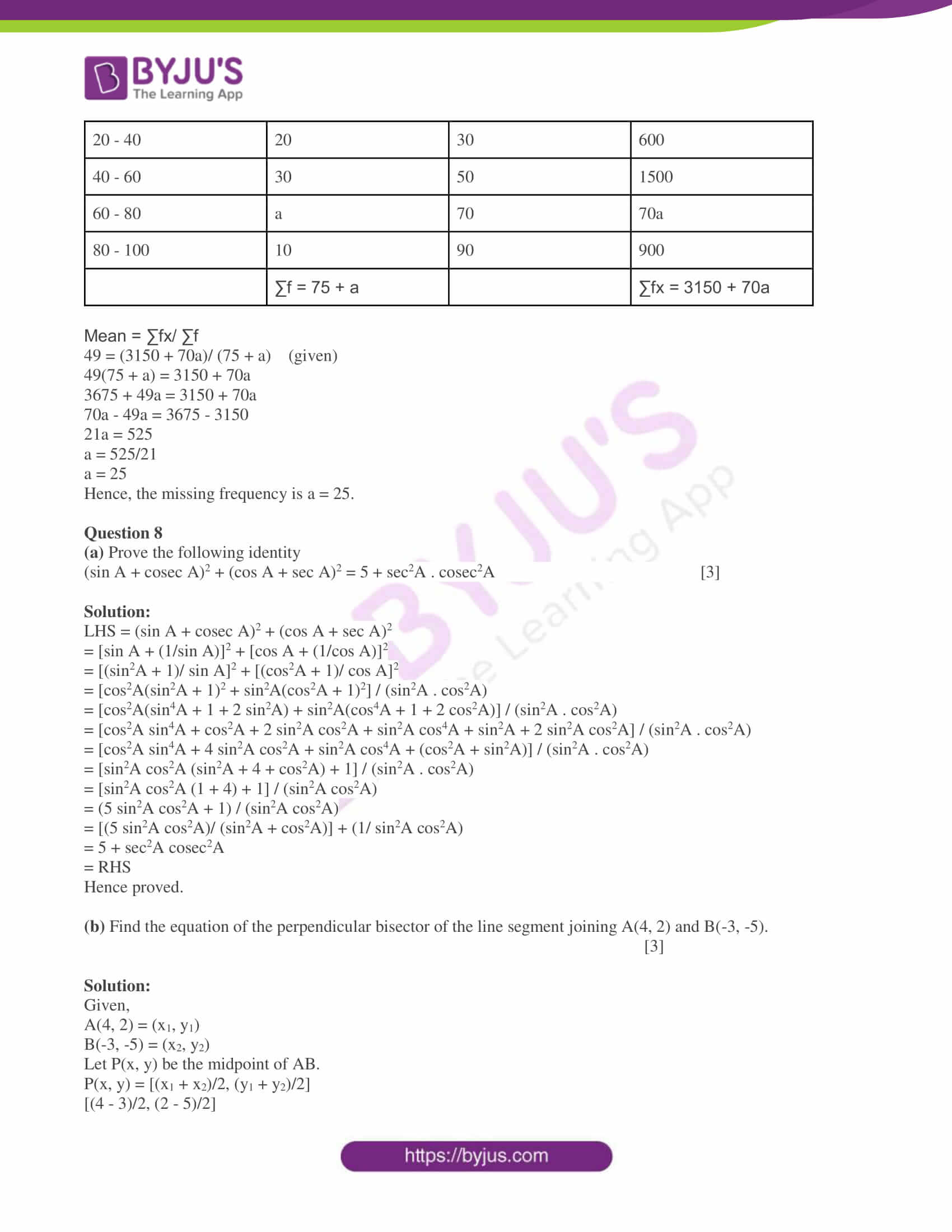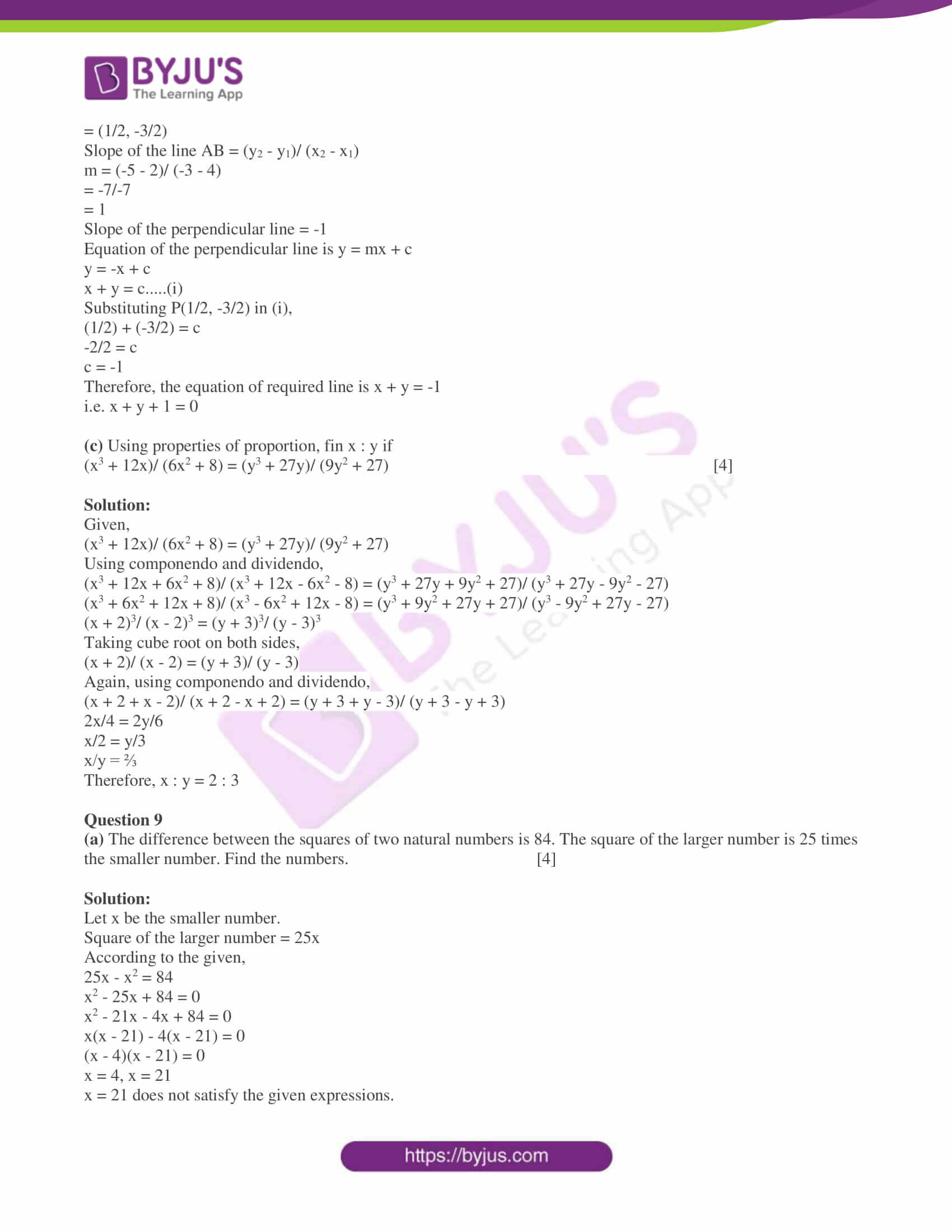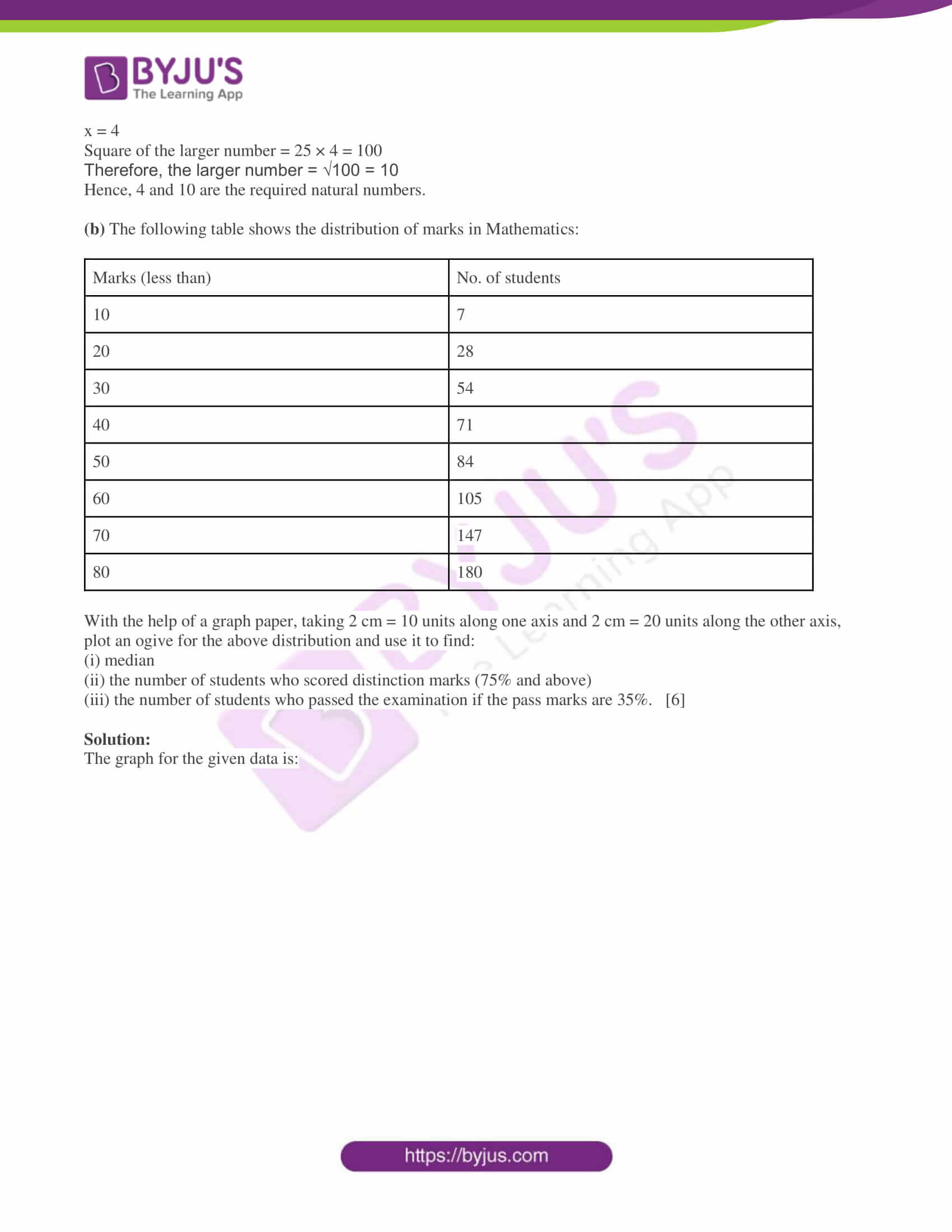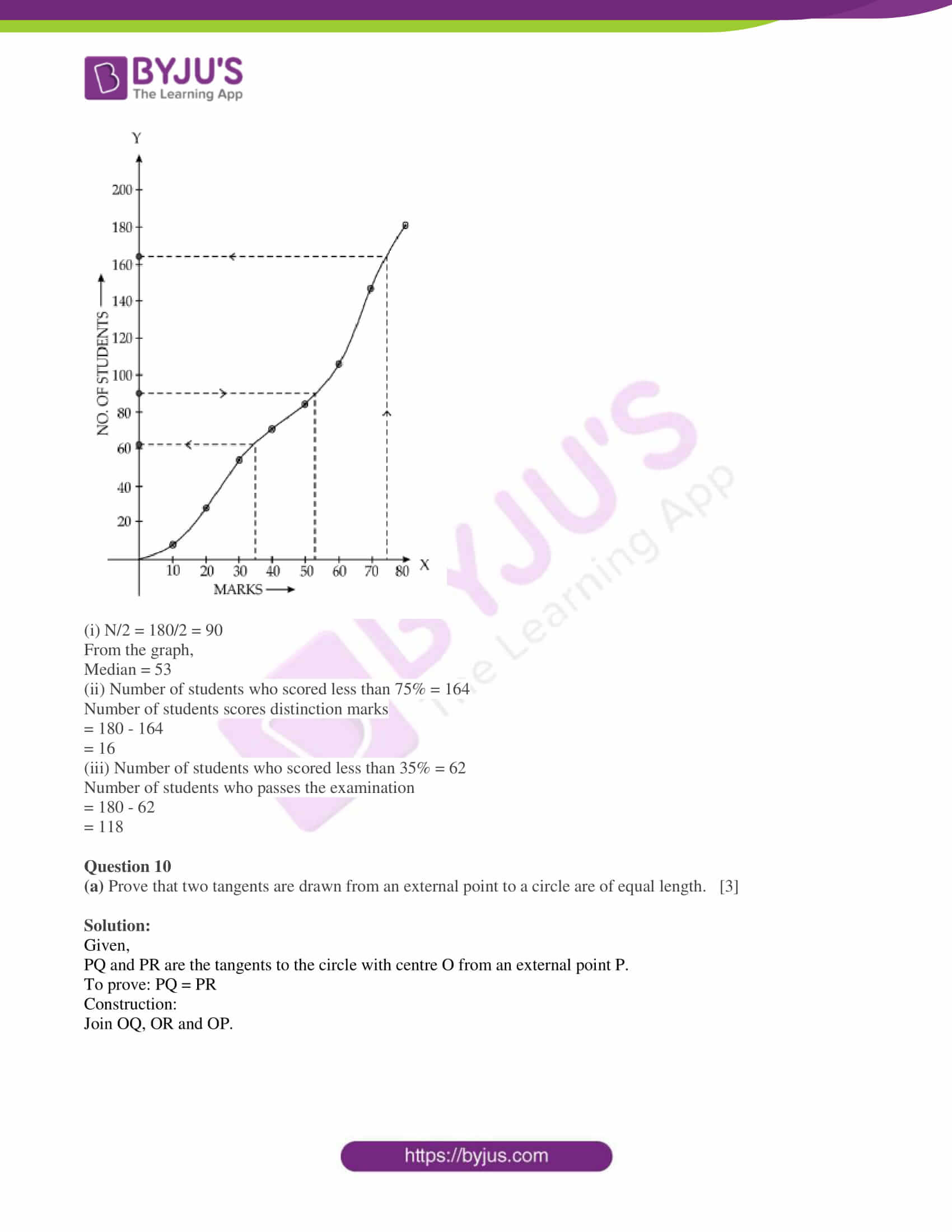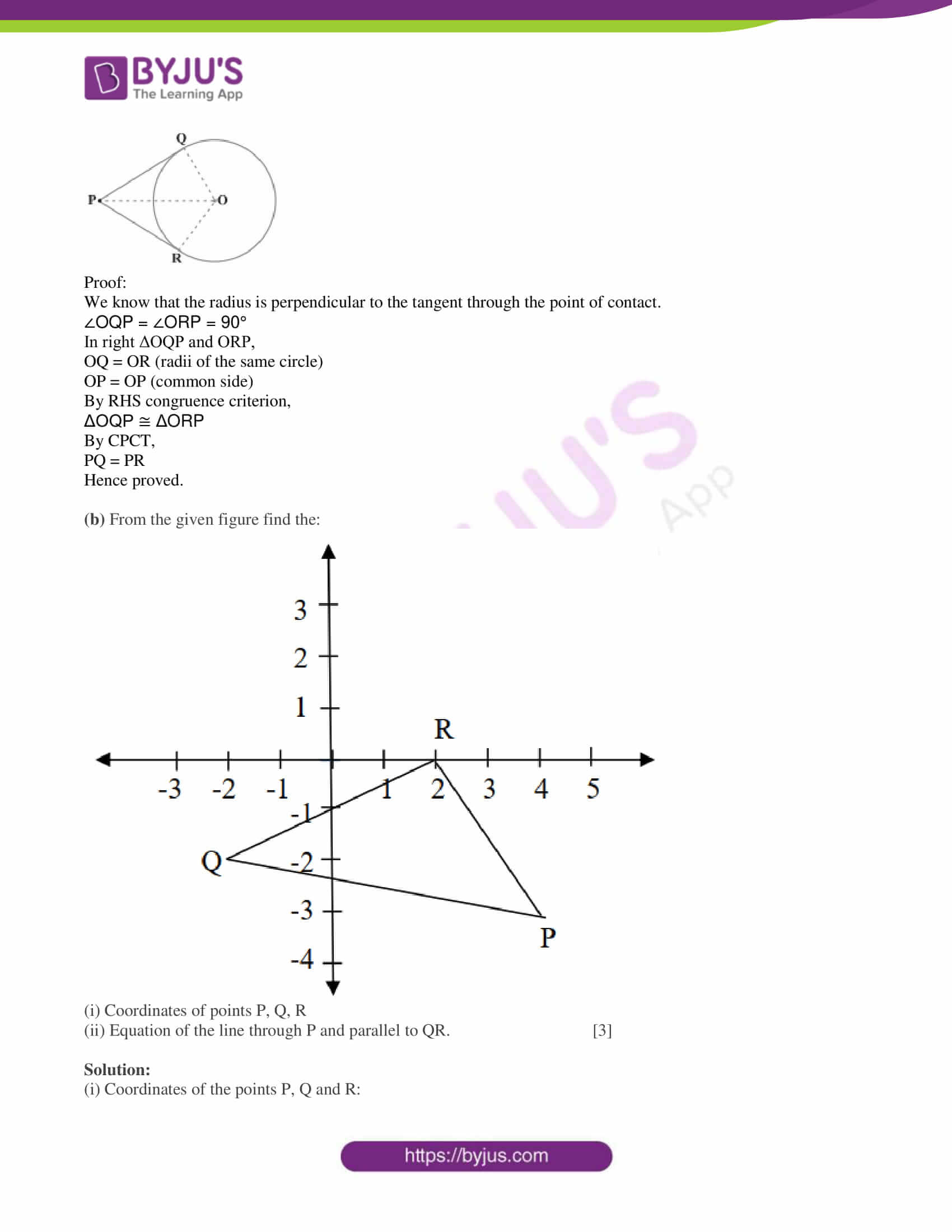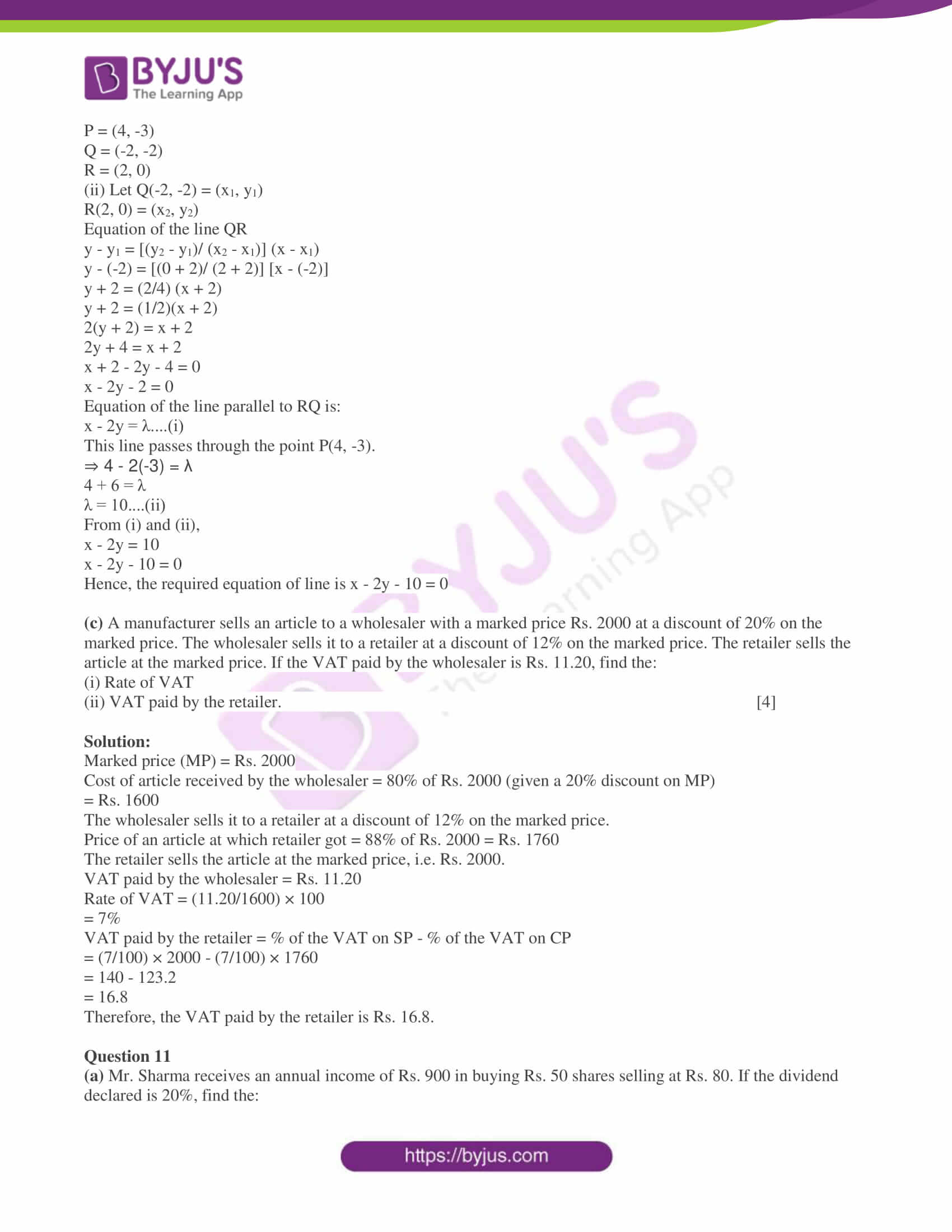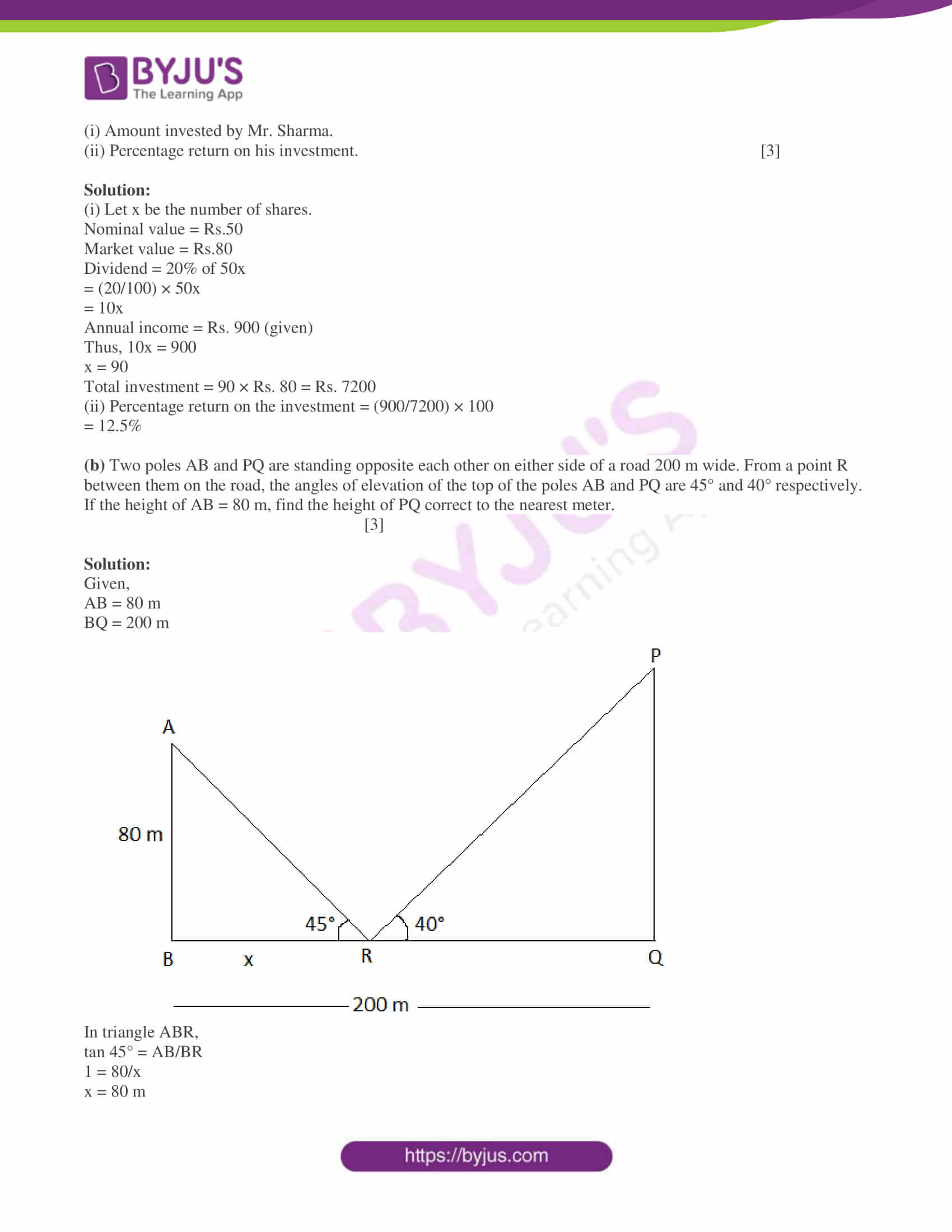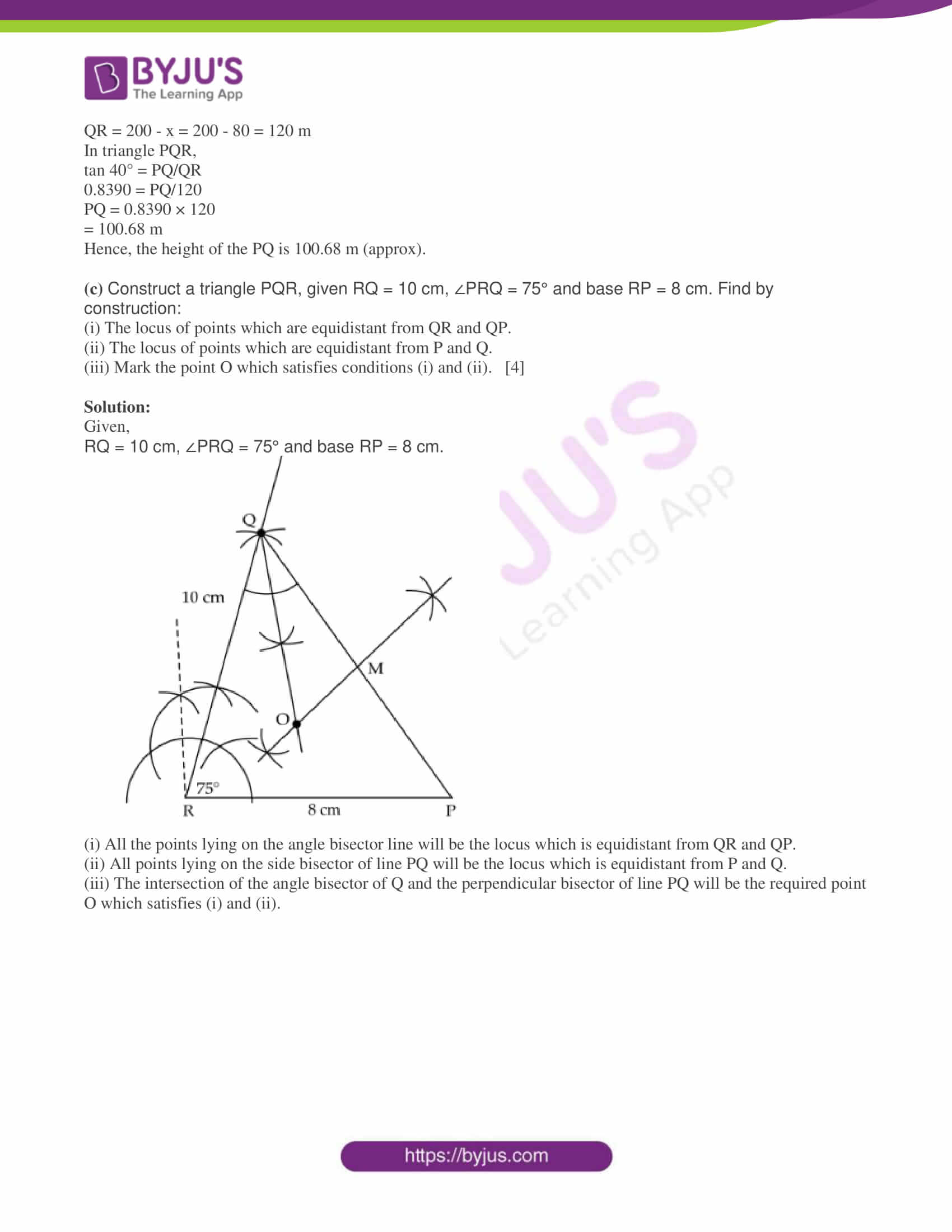SECTION A

Attempt all questions from this Section.

Question 1

(a) Find the value of ‘k’ if 4x3 – 2x2 + kx + 5 leaves remainder -10 when divided by 2x + 1. 

Solution:

Let p(x) = 4x3 – 2x2 + kx + 5

g(x) = 2x + 1

r(x) = -10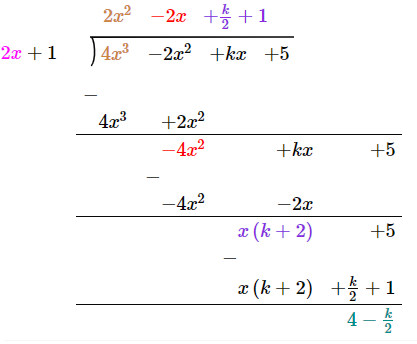4 – (k/2) = -10 (given)

-k/2 = -10 – 4

-k/2 = -14

k = 14(2)

k = 28

(b) Amit deposits Rs. 1600 per month in a bank for 18 months in a recurring deposit account. If he gets Rs. 31,080 at the time of maturity, what is the rate of interest per annum? 

Solution:

Given,

P = Rs. 1600

n = 18

MV = Rs. 31,080

Let R be the rate of interest.

MV = P × n + I

I = MV – P × n

= 31080 – 1600 18

= 31080 – 28800

= 2280

I = [n(n + 1)/2] × [(P × R)/ 100 × 12]

2280 = [(18 × 19)/2] × [(1600 × R)/ 1200]

2280 = 171 × (4/3) × R

R = (2280 × 3)/ (171 × 4)

R = 6840/684

R = 10%

(c) The price of an article is Rs. 9350 which includes VAT at 10%. Find how much less a customer pays for the article if the VAT on the article decreases by 3%. 

Solution:

Price of an article including 10% VAT = Rs. 9350

i.e. 110% = 9350

Original price = (100/110) × Rs. 9350

= Rs. 8500

3% of Rs. 8500 = (3/100) × Rs. 8500

= Rs. 255

Hence, the customer pays Rs. 255 less for the article if VAT decreases by 3%.

Question 2

(a) Solve the following inequation and represent your solution on the real number line:

-5 1/2 – x ≤ 1/2 – 3x ≤ 3 1/2 – x, x ∈ R 

Solution:

-5 1/2 – x ≤ 1/2 – 3x ≤ 3 1/2 – x

-11/2 – x ≤ (1 – 6x)/2 ≤ 7/2 – x

(-11 – 2x)/2 ≤ (1 – 6x)/2 ≤ (7 – 2x)/2

–11 – 2x) ≤ (1 – 6x) ≤ (7 – 2x)

Now,

-11 – 2x ≤ 1 – 6x

-2x + 6x ≤ 1 + 11

4x ≤ 12

x ≤ 3

And

1 – 6x ≤ 7 – 2x

-6x + 2x ≤ 7 – 1

-4x ≤ 6

-x ≤ 3/2

x ≥ -3/2

Therefore, 3 ≥ x ≥ -3/2

Or

-3/2 ≤ x ≤ 3

This can be represented on the number line as: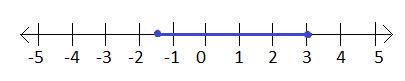(b) Find the 16th term of the A.P. 7, 11, 15, 19,… Find the sum of the first 6 terms. 

Solution:

Given,

7, 11, 15, 19,…

First term = a = 7

Common difference = d = 11 – 7 = 4

nth term of an AP:

an = a + (n – 1)d

a16 = a + (16 – 1)d

= 7 + 15(4)

= 7 + 60

= 67

Therefore, the 16th term of the AP is 67.

Sum of first n terms of an AP:

Sn = n/2 [2a + (n – 1)d]

S6 = (6/2) [2(7) + (6 – 1)4]

= 3 [14 + (5)4]

= 3 [14 + 20]

= 3 × 34

= 102

Hence, the sum of the first 6 terms is 102.

(c) In the given figure, CE is a tangent to the circle at point C. ABCD is a cyclic quadrilateral. If ∠ABC = 93° and ∠DCE = 35°.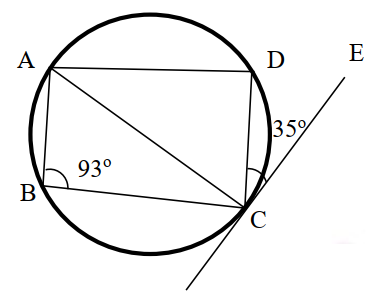Find:

(iii) ∠ACD 

Solution:

Given,

CE is a tangent to the circle at point C.

∠ABC = 93° and ∠DCE = 35°

(i) We know that the sum of the opposite angles of a cyclic quadrilateral is 180°.

The angle subtended by a chord in an alternate segment of the circle.

By the angle sum property:

∠ACD + 35° + 87° = 180°

∠ACD = 180° – 122°

∠ACD = 58°

Question 3

(a) Prove the following identity

[sec A/ (sec A – 1)] + [sec A/ (sec A + 1)] = 2 cosec2A 

Solution:

LHS = [sec A/ (sec A – 1)] + [sec A/ (sec A + 1)]

= [sec A(sec A + 1) + sec A(sec A – 1)] / [(sec A – 1)(sec A + 1)]

= [sec2A + sec A + sec2A – sec A]/ (sec2A – 1)

= 2 sec2A/ tan2A

= 2 cos2A/ (sin2A cos2A)

= 2/sin2A

= 2 cosec2A

= RHS

Hence proved.

(b) Find x and y if: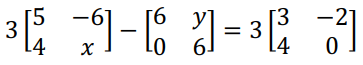Solution:

Given,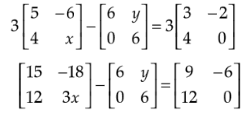⇒ 3x – 6 = 0

⇒ 3x = 6

⇒ x = 6/3 = 2

And

-18 – y = -6

-18 + 6 = y

⇒ y = -12

Therefore, x = 2 and y = -12.

(c) For what value of ‘k’ will the following quadratic equation:

(k + 1)x2 – 4kx + 9 = 0 have real and equal roots? Solve the equations. 

Solution:

Given,

(k + 1)x2 – 4kx + 9 = 0

Comparing with the standard form ax2 + bx + c = 0,

a = (k + 1), b = -4k, c = 9

Since, the given quadratic equation has real and equal roots, discriminant = 0

b2 – 4ac = 0

(-4k)2 – 4(k + 1)(9) = 0

16k2 – 36k – 36 = 0

4(4k2 – 9k – 9) = 0

4k2 – 9k – 9 = 0

4k2 – 12k + 3k – 9 = 0

4k(k – 3) + 3(k – 3) = 0

(4k + 3)(k – 3) = 0

4k + 3 = 0, k – 3 = 0

k = -3/4, k = 3

When k = -3/4,

[(-3/4) + 1]x2 – 4(-3/4)x + 9 = 0

(1/4)x2 + 3x + 9 = 0

⇒ x2 + 12x + 36 = 0

⇒ x2 + 6x + 6x + 36 = 0

⇒ x(x + 6) + 6(x + 6) = 0

⇒ (x + 6)(x + 6) = 0

⇒ x = -6, x = -6

When k = 3,

(3 + 1)x2 – 4(3)x + 9 = 0

4x2 – 12x + 9 = 0

4x2 – 6x – 6x + 9 = 0

2x(2x – 3) – 3(2x – 3) = 0

(2x – 3)(2x – 3) = 0

⇒ x = 3/2, x = 3/2

Question 4

(a) A box consists of 4 red, 5 black, and 6 white balls. One ball is drawn out at random. Find the probability that the ball drawn is:

(i) black

(ii) red or white 

Solution:

Given,

A box consists of 4 red, 5 black, and 6 white balls.

Total number of outcomes = 4 + 5 + 6 = 15

(i) Number of black balls = 5

P(getting the black ball) = 5/15 = ⅓

(ii) Number of red and white balls = 4 + 6 = 10

P(getting a red or white ball) = 10/15 = ⅔

(b) Calculate the median and mode for the following distribution:

 Weight (in kg) 35 47 52 56 60 No. of students 4 3 5 3 2


Solution:

 Weight (in kg) x No. of students (f) Cumulative frequency (cf) 35 4 4 47 3 7 52 5 12 56 3 15 60 2 17 N = 17

N/2 = 17/2 = 8.5

Cumulative frequency greater than and nearest to 8.5 is 12.

Therefore, median = 52

Highest frequency = 5

Hence, the mode = 52

(c) A solid cylinder of radius 7 cm and height 14 cm is melted and recast into solid spheres each of radius 3.5 cm. Find the number of spheres formed. 

Solution:

Given,

Radius of cylinder = R = 7 cm

Height of cylinder = H = 14 cm

Radius of sphere = r = 3.5 cm

Let n be the number of spheres formed.

Volume of cylinder = n × Volume of one sphere

πR2H = n × (4/3)πr3

7 × 7 × 14 = n × (4/3) × 3.5 × 3.5 × 3.5

n = (3 × 7 × 7 × 14)/ (4 × 3.5 × 3.5 × 3.5)

= 12

Hence, 12 spheres are formed by melting the cylinder.

SECTION B

Attempt any four questions from this Section.

Question 5

(a) The 2nd and 45th terms of an arithmetic progression are 10 and 96 respectively. Find the first term and the common difference and hence find the sum of the first 15 terms. 

Solution:

Let a be the first term and d be the common difference of an AP.

nth term of an AP:

an = a + (n – 1)d

Given,

a2 = 10

a + d = 10…..(i)

And

a45 = 96

a + 44d = 96….(ii)

Subtracting (i) from (ii),

a + 44d – (a + d) = 96 – 10

44d – d = 86

43d = 86

d = 86/43

d = 2

Substituting d = 2 in (i),

a + 2 = 10

a = 10 – 2

a = 8

Sum of first n terms:

Sn = n/2 [2a + (n – 1)d]

S15 = (15/2) [2(8) + (15 – 1)2]

= (15/2) [16 + (14)2]

= (15/2) [16 + 28]

= (15/2) × 44

= 330

Therefore, the sum of the first 15 terms is 330.

(b)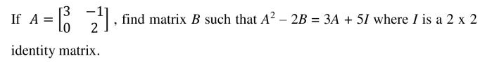Solution:

Given,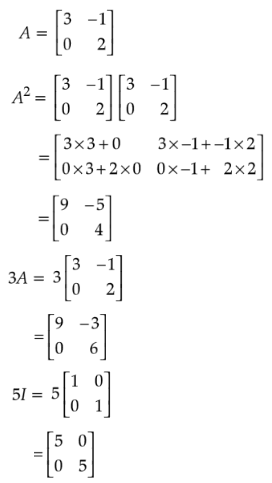Also,

A2 – 2B = 3A + 5I

2B = A2 – 3A – 5I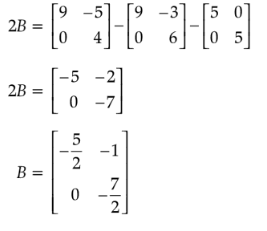(c) With the help of a graph paper, taking 1 cm = 1 unit along both x and y-axis:

(i) Plot points A(0, 3), B(2, 3), C(3, 0), D(2, -3), E(0, -3)

(ii) Reflect points B, C, and D on the y-axis and name them as B’, C’, and D’ respectively.

(iii) Write the coordinates of B’, C’, and D’.

(iv) Write the equation of line B’D’.

(v) Name the figure BCDD’C’B’B 

Solution:

(i) A(0, 3), B(2, 3), C(3, 0), D(2, -3), E(0, -3)

(ii) Reflect points B, C, and D on the y-axis namely B’, C’, and D’ respectively.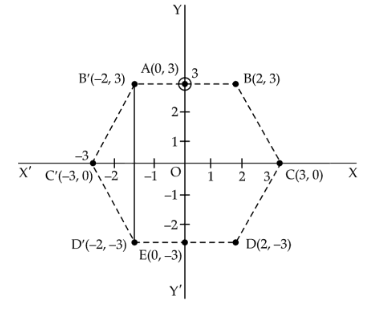(iii) Coordinates of B’, C’, and D’:

B'(-2, 3), C'(-3, 0) and D'(-2, -3).

(iv) Equation of line B’D’

y – 3 = [(-3 – 3)/ (-2 + 2)] [x – (-2)]

y – 3 = (-6/0) (x + 2)

⇒ -6(x + 2) = 0

⇒ x + 2 = 0

(v) Name of the figure BCDD’C’B’B is Hexagon.

Question 6

(a) In ΔABC and ΔEDC, AB is parallel to ED. BD = 1/3 BC and AB = 12.3 cm.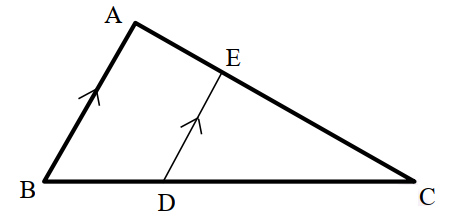(i) Prove that ΔABC ~ ΔEDC.

(ii) Find DE

(iii) Find: (area of ΔEDC)/ (area of ΔABC) 

Solution:

(i) In ΔABC and ΔDEC,

AB || ED

∠ABC = ∠EDC (corresponding angles)

∠BAC = ∠DEC (corresponding angles)

∠C = ∠C (common)

By AA similarity criterion,

ΔABC ~ ΔEDC

(ii) By Thales theorem,

BC/CD = AB/ED

BC/ [(2/3) BC] = 12.3/ED

3/2 = 12.3/ED

ED = (12.3 × 2)/3

ED = 8.2 cm

(iii) (area of ΔEDC)/ (area of ΔABC) = DE2/ AB2

= (8.2)2/ (12.3)2

= 67.24/151.29

= 6724/ 15129

(b) Find the ratio in which the line joining (-2, 5) and (-5, -6) is divided by the line y = -3. Hence find the point of intersection. 

Solution:

Let the line y = -3 divides the line joining the points A(-2, 5) and B(-5, -6) at the point P(x, y) in the ratio λ : 1

A(-2, 5) = (x1, y1)

B(-5, -6) = (x2, y2)

Using the section formula,

x = (λx2 + x1)/ (λ + 1)

= (-5λ – 2)/ (λ + 1)

And

y = (λy2 + y1)/ (λ + 1)

= (-6λ + 5)/ (λ + 1)

P(x, y) = [(-5λ – 2)/(λ + 1), (-6λ + 5)/(λ + 1)]

P lies on the line y = -3

(-6λ + 5)/(λ + 1) = -3

-6λ + 5 = -3λ – 3

3λ = 8

λ = 8/3

Therefore, the required ratio is 8 : 3.

x = (-5λ – 2)/ (λ + 1)

= [-5(8/3) – 2]/ [(8/3) + 1]

= [(-40 – 6)/3] × [3/ (8 + 3)]

= -46/11

y = (-6λ + 5)/ (λ + 1)

= [-6(8/3) + 5]/ [(8/3) + 1]

= [(-48 + 15)/3] × [3/ (8 + 3)]

= -33/11

= -3

Hence, the point of intersection is P(-46/11, -3)

(c) The given solid figure is a cylinder surmounted by a cone. The diameter of the base of the cylinder is 6 cm. The height of the cone is 4 cm and the total height of the solid is 25 cm. Take π = 22/7.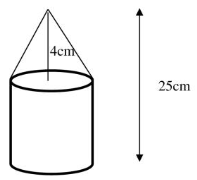Find the:

(i) Volume of the solid

(ii) Curved surface area of the solid

Solution:

Given,

Diameter of the base of the cylinder = 6 cm

Radius of cylinder = Radius of cone = r = 6/2 = 3 cm

Height of the cone = h = 4 cm

Total height of the solid = 25 cm

Height of the cylinder = H = 25 – 4 = 21 cm

Slant height of cone = √(r2 + h2)

l = √(32 + 42)

= √(9 + 16)

= √25

= 5 cm

(i) Volume of the solid

= Volume of cylinder + Volume of cone

= πr2H + (1/3) πr2h

= πr2(H + h/3)

= (22/7) × 3 × 3 × [21 + (4/3)]

= (22/7) × 9 × (67/3)

= 631.72

= 632 cm3 (approx)

(ii) Curved surface area of the solid

= CSA of cylinder + CSA of cone

= 2πrH + πrl

= πr(2H + l)

= (22/7) × 3 × (2 × 21 + 5)

= (22/7) × 3 × 47

= 443.14

= 443 cm2 (approx)

Question 7

(a) In the given figure, PAB is secant and PT is a tangent to the circle with centre O. If ∠ATP = 40°, PA = 9 cm, and AB = 7 cm.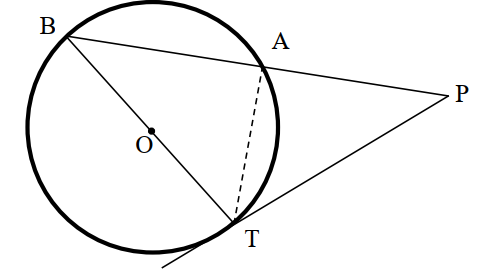Find:

(i) ∠APT

(ii) length of PT 

Solution:

(i) ∠BAT = 90° (angle in the semicircle)

∠BAT + ∠PAT = 180° (linear pair)

90° + ∠PAT = 180°

∠PAT = 180° – 90°

∠PAT = 90°

In triangle PAT,

∠APT + ∠ATP + ∠PAT = 180° (by the angle sum property of a triangle)

∠APT + 40° + 90° = 180°

∠APT = 180° – (40° + 90°)

∠APT = 50°

(ii) PB = PA + AB

= 9 + 7

= 16 cm

Also, PT2 = PB × PA

= 16 × 9

= 144

PT = √144

PT = 12 cm

(b) The 1st and the 8th term of a GP are 4 and 512 respectively. Find:

(i) the common ratio

(ii) the sum of its first 5 terms. 

Solution:

Given,

First term of a GP = a = 4

8th term = t8 = 512

(i) ar(8 – 1) = 512

4r7 = 512

r7 = 512/4

r7 = 128

r7 = (2)7

⇒ r = 2

Common ratio = 2

(ii) Sum of the first n terms:

Sn = a(rn – 1)/ (r – 1)

S5 = [4(25 – 1)]/ (2 – 1)

= 4(32 – 1)/1

= 4 × 31

= 124

Therefore, the sum of the first 5 terms of the GP is 124.

(c) The mean of the following distribution is 49. Find the missing frequency ‘a’.

 Class 0 – 20 20 – 40 40 – 60 60 – 80 80 – 100 Frequency 15 20 30 a 10


Solution:

 Class Frequency (f) Class mark (x) fx 0 – 20 15 10 150 20 – 40 20 30 600 40 – 60 30 50 1500 60 – 80 a 70 70a 80 – 100 10 90 900 ∑f = 75 + a ∑fx = 3150 + 70a

Mean = ∑fx/ ∑f

49 = (3150 + 70a)/ (75 + a) (given)

49(75 + a) = 3150 + 70a

3675 + 49a = 3150 + 70a

70a – 49a = 3675 – 3150

21a = 525

a = 525/21

a = 25

Hence, the missing frequency is a = 25.

Question 8

(a) Prove the following identity

(sin A + cosec A)2 + (cos A + sec A)2 = 5 + sec2A . cosec2A 

Solution:

LHS = (sin A + cosec A)2 + (cos A + sec A)2

= [sin A + (1/sin A)]2 + [cos A + (1/cos A)]2

= [(sin2A + 1)/ sin A]2 + [(cos2A + 1)/ cos A]2

= [cos2A(sin2A + 1)2 + sin2A(cos2A + 1)2] / (sin2A . cos2A)

= [cos2A(sin4A + 1 + 2 sin2A) + sin2A(cos4A + 1 + 2 cos2A)] / (sin2A . cos2A)

= [cos2A sin4A + cos2A + 2 sin2A cos2A + sin2A cos4A + sin2A + 2 sin2A cos2A] / (sin2A . cos2A)

= [cos2A sin4A + 4 sin2A cos2A + sin2A cos4A + (cos2A + sin2A)] / (sin2A . cos2A)

= [sin2A cos2A (sin2A + 4 + cos2A) + 1] / (sin2A . cos2A)

= [sin2A cos2A (1 + 4) + 1] / (sin2A cos2A)

= (5 sin2A cos2A + 1) / (sin2A cos2A)

= [(5 sin2A cos2A)/ (sin2A + cos2A)] + (1/ sin2A cos2A)

= 5 + sec2A cosec2A

= RHS

Hence proved.

(b) Find the equation of the perpendicular bisector of the line segment joining A(4, 2) and B(-3, -5). 

Solution:

Given,

A(4, 2) = (x1, y1)

B(-3, -5) = (x2, y2)

Let P(x, y) be the midpoint of AB.

P(x, y) = [(x1 + x2)/2, (y1 + y2)/2] [(4 – 3)/2, (2 – 5)/2]

= (1/2, -3/2)

Slope of the line AB = (y2 – y1)/ (x2 – x1)

m = (-5 – 2)/ (-3 – 4)

= -7/-7

= 1

Slope of the perpendicular line = -1

Equation of the perpendicular line is y = mx + c

y = -x + c

x + y = c…..(i)

Substituting P(1/2, -3/2) in (i),

(1/2) + (-3/2) = c

-2/2 = c

c = -1

Therefore, the equation of required line is x + y = -1

i.e. x + y + 1 = 0

(c) Using properties of proportion, fin x : y if

(x3 + 12x)/ (6x2 + 8) = (y3 + 27y)/ (9y2 + 27) 

Solution:

Given,

(x3 + 12x)/ (6x2 + 8) = (y3 + 27y)/ (9y2 + 27)

Using componendo and dividendo,

(x3 + 12x + 6x2 + 8)/ (x3 + 12x – 6x2 – 8) = (y3 + 27y + 9y2 + 27)/ (y3 + 27y – 9y2 – 27)

(x3 + 6x2 + 12x + 8)/ (x3 – 6x2 + 12x – 8) = (y3 + 9y2 + 27y + 27)/ (y3 – 9y2 + 27y – 27)

(x + 2)3/ (x – 2)3 = (y + 3)3/ (y – 3)3

Taking cube root on both sides,

(x + 2)/ (x – 2) = (y + 3)/ (y – 3)

Again, using componendo and dividendo,

(x + 2 + x – 2)/ (x + 2 – x + 2) = (y + 3 + y – 3)/ (y + 3 – y + 3)

2x/4 = 2y/6

x/2 = y/3

x/y = ⅔

Therefore, x : y = 2 : 3

Question 9

(a) The difference between the squares of two natural numbers is 84. The square of the larger number is 25 times the smaller number. Find the numbers. 

Solution:

Let x be the smaller number.

Square of the larger number = 25x

According to the given,

25x – x2 = 84

x2 – 25x + 84 = 0

x2 – 21x – 4x + 84 = 0

x(x – 21) – 4(x – 21) = 0

(x – 4)(x – 21) = 0

x = 4, x = 21

x = 21 does not satisfy the given expressions.

x = 4

Square of the larger number = 25 × 4 = 100

Therefore, the larger number = √100 = 10

Hence, 4 and 10 are the required natural numbers.

(b) The following table shows the distribution of marks in Mathematics:

 Marks (less than) No. of students 10 7 20 28 30 54 40 71 50 84 60 105 70 147 80 180

With the help of a graph paper, taking 2 cm = 10 units along one axis and 2 cm = 20 units along the other axis, plot an ogive for the above distribution and use it to find:

(i) median

(ii) the number of students who scored distinction marks (75% and above)

(iii) the number of students who passed the examination if the pass marks are 35%. 

Solution:

The graph for the given data is: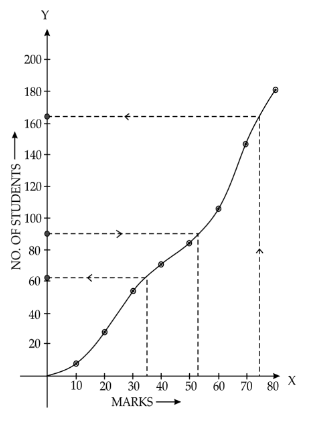(i) N/2 = 180/2 = 90

From the graph,

Median = 53

(ii) Number of students who scored less than 75% = 164

Number of students scores distinction marks

= 180 – 164

= 16

(iii) Number of students who scored less than 35% = 62

Number of students who passes the examination

= 180 – 62

= 118

Question 10

(a) Prove that two tangents are drawn from an external point to a circle are of equal length. 

Solution:

Given,

PQ and PR are the tangents to the circle with centre O from an external point P.

To prove: PQ = PR

Construction:

Join OQ, OR and OP.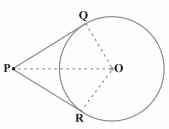Proof:

We know that the radius is perpendicular to the tangent through the point of contact.

∠OQP = ∠ORP = 90°

In right ΔOQP and ORP,

OQ = OR (radii of the same circle)

OP = OP (common side)

By RHS congruence criterion,

ΔOQP ≅ ΔORP

By CPCT,

PQ = PR

Hence proved.

(b) From the given figure find the: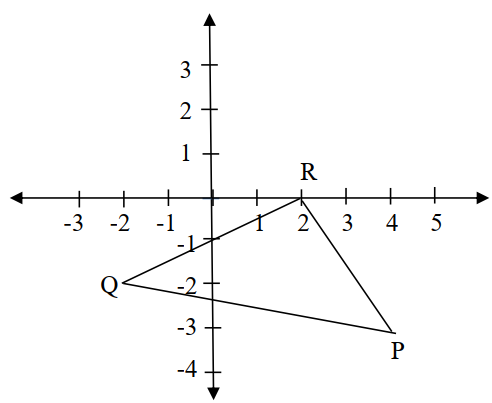(i) Coordinates of points P, Q, R

(ii) Equation of the line through P and parallel to QR. 

Solution:

(i) Coordinates of the points P, Q and R:

P = (4, -3)

Q = (-2, -2)

R = (2, 0)

(ii) Let Q(-2, -2) = (x1, y1)

R(2, 0) = (x2, y2)

Equation of the line QR

y – y1 = [(y2 – y1)/ (x2 – x1)] (x – x1)

y – (-2) = [(0 + 2)/ (2 + 2)] [x – (-2)]

y + 2 = (2/4) (x + 2)

y + 2 = (1/2)(x + 2)

2(y + 2) = x + 2

2y + 4 = x + 2

x + 2 – 2y – 4 = 0

x – 2y – 2 = 0

Equation of the line parallel to RQ is:

x – 2y = λ….(i)

This line passes through the point P(4, -3).

⇒ 4 – 2(-3) = λ

4 + 6 = λ

λ = 10….(ii)

From (i) and (ii),

x – 2y = 10

x – 2y – 10 = 0

Hence, the required equation of line is x – 2y – 10 = 0

(c) A manufacturer sells an article to a wholesaler with a marked price Rs. 2000 at a discount of 20% on the marked price. The wholesaler sells it to a retailer at a discount of 12% on the marked price. The retailer sells the article at the marked price. If the VAT paid by the wholesaler is Rs. 11.20, find the:

(i) Rate of VAT

(ii) VAT paid by the retailer. 

Solution:

Marked price (MP) = Rs. 2000

Cost of article received by the wholesaler = 80% of Rs. 2000 (given a 20% discount on MP)

= Rs. 1600

The wholesaler sells it to a retailer at a discount of 12% on the marked price.

Price of an article at which retailer got = 88% of Rs. 2000 = Rs. 1760

The retailer sells the article at the marked price, i.e. Rs. 2000.

VAT paid by the wholesaler = Rs. 11.20

Rate of VAT = (11.20/1600) × 100

= 7%

VAT paid by the retailer = % of the VAT on SP – % of the VAT on CP

= (7/100) × 2000 – (7/100) × 1760

= 140 – 123.2

= 16.8

Therefore, the VAT paid by the retailer is Rs. 16.8.

Question 11

(a) Mr. Sharma receives an annual income of Rs. 900 in buying Rs. 50 shares selling at Rs. 80. If the dividend declared is 20%, find the:

(i) Amount invested by Mr. Sharma.

(ii) Percentage return on his investment. 

Solution:

(i) Let x be the number of shares.

Nominal value = Rs.50

Market value = Rs.80

Dividend = 20% of 50x

= (20/100) × 50x

= 10x

Annual income = Rs. 900 (given)

Thus, 10x = 900

x = 90

Total investment = 90 × Rs. 80 = Rs. 7200

(ii) Percentage return on the investment = (900/7200) × 100

= 12.5%

(b) Two poles AB and PQ are standing opposite each other on either side of a road 200 m wide. From a point R between them on the road, the angles of elevation of the top of the poles AB and PQ are 45° and 40° respectively. If the height of AB = 80 m, find the height of PQ correct to the nearest meter. 

Solution:

Given,

AB = 80 m

BQ = 200 m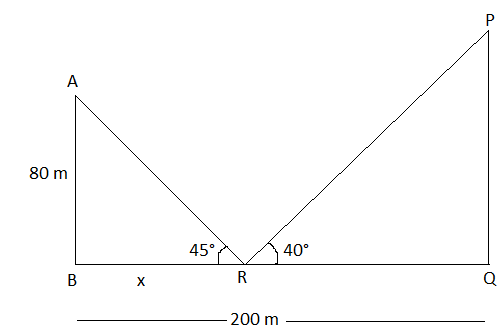In triangle ABR,

tan 45° = AB/BR

1 = 80/x

x = 80 m

QR = 200 – x = 200 – 80 = 120 m

In triangle PQR,

tan 40° = PQ/QR

0.8390 = PQ/120

PQ = 0.8390 × 120

= 100.68 m

Hence, the height of the PQ is 100.68 m (approx).

(c) Construct a triangle PQR, given RQ = 10 cm, ∠PRQ = 75° and base RP = 8 cm. Find by construction:

(i) The locus of points which are equidistant from QR and QP.

(ii) The locus of points which are equidistant from P and Q.

(iii) Mark the point O which satisfies conditions (i) and (ii). 

Solution:

Given,

RQ = 10 cm, ∠PRQ = 75° and base RP = 8 cm.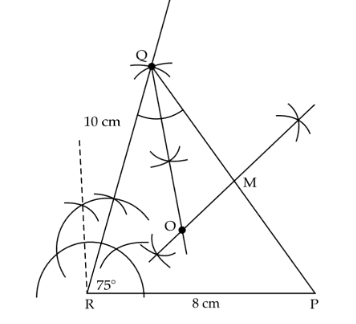(i) All the points lying on the angle bisector line will be the locus which is equidistant from QR and QP.

(ii) All points lying on the side bisector of line PQ will be the locus which is equidistant from P and Q.

(iii) The intersection of the angle bisector of Q and the perpendicular bisector of line PQ will be the required point O which satisfies (i) and (ii).